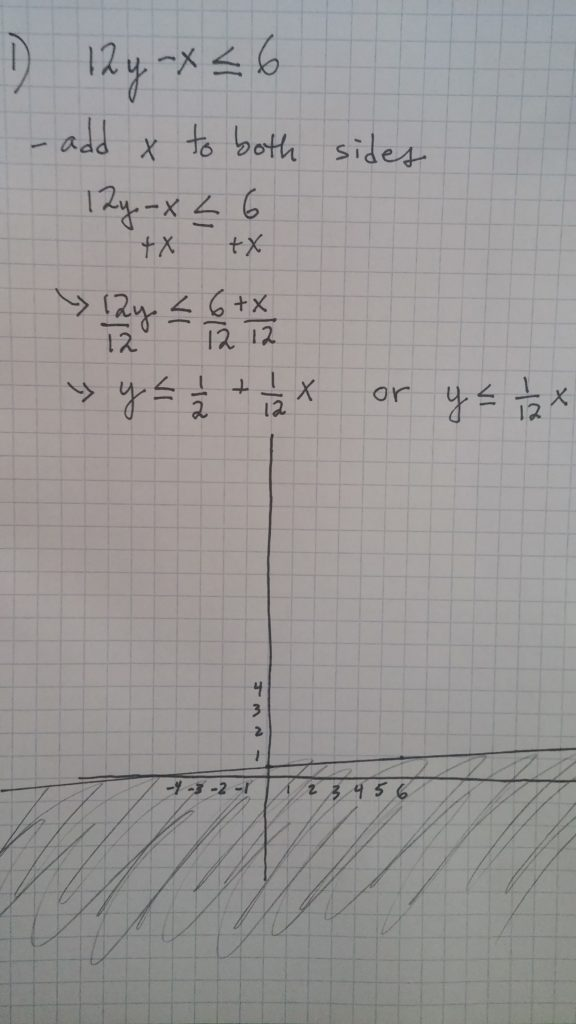# Graphin inequalities

Take an question such as: Graph 12y-x ≤ 6 on a coordinate plane. First, recall y=mx+b, which describes linear functions. We want to rearrange 12y-x≤6 so that it closely resembles that form. Start by adding x to both sides so that you have: 12y ≤ x+6. Now, divide all terms by 12 so that you get y ≤ (1/12)x+1/2. Now graph the line y=(1/12)x+1/2 as a solid line (because this is “less than or equal to.” If it was simply “less than” then we would do a dashed line.Now that you have draw that line on the coordinate plane, go ahead and shade the region below that line. This is because we are considering all values “less than or equal to” as opposed to simply y=(1/12)x+1/2, which we represent with just a line. That’s it!